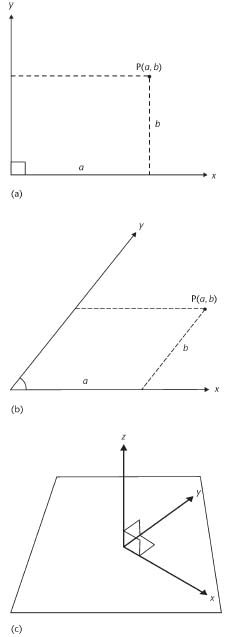Definition: Cartesian coordinates from Collins English Dictionary

pl n

1 a system of representing points in space in terms of their distance from a given origin measured along a set of mutually perpendicular axes. Written (x,y,z) with reference to three axes

Summary Article: Cartesian coordinate system
From The Penguin Dictionary of Mathematics

A coordinate system in which the position of a point is determined by its relation to reference lines (axes). In two dimensions, two lines are used; commonly the lines are at right angles, forming a rectangular coordinate system (see diagram (a)). The horizontal axis is the x-axis and the vertical axis is the y-axis. The point of intersection O is the origin of the coordinate system. Distances along the x-axis to the right of the origin are usually taken as positive, distances to the left negative. Distances along the y-axis above the origin are positive; distances below are negative. The position of a point anywhere in the plane can then be specified by two numbers, the coordinates of the point, written as (x,y). The x-coordinate (or abscissa) is the distance of the point from the y-axis in a direction parallel to the x-axis (i.e. horizontally). The y-coordinate (or ordinate) is the distance from the x-axis in a direction parallel to the y-axis (vertically). The origin O is the point (0, 0). The two axes divide the plane into four quadrants, numbered anticlockwise starting from the top right (positive) quadrant: the first quadrant.

Cartesian coordinates were first introduced in the 17th century by Rene Descartes. Their discovery allowed the application of algebraic methods to geometry and the study of hitherto unknown curves. As a point in Cartesian coordinates is represented by an ordered pair of numbers, so is a line represented by an equation. Thus, y = x represents a set of points for which the x-coordinate equals the y-coordinate; i.e. y = x is a straight line through the origin at 45° to the axes. Equations of higher degree represent curves; for example,

x2 + y2=4

is a circle of radius 2 with its centre at the origin. A curve drawn in a Cartesian coordinate system for a particular equation or function is a graph of the equation or function.

The axes in a planar Cartesian coordinate system need not necessarily be at right angles to each other. If the x- and y-axes make an angle other than 90° the system is said to be an oblique coordinate system (see diagram (b)). Distances from the axes are then measured along lines parallel to the axes.

Cartesian coordinate systems can also be used for three dimensions by including a third axis - the z-axis - through the origin perpendicular to the other two. The position of a point is then given by three coordinates (x, y, z). The coordinate axes may be left-handed or right-handed, depending on the way positive directions are given to the axes. In a right-handed system (see diagram (c)), if the thumb of the right hand points in the positive direction of the x-axis, the first and second fingers can be pointed in the positive directions of the y- and z-axes respectively. The axes are said to form a right-handed triad. A left-handed system is the mirror image of this (i.e. determined using the left hand), the axes being said to form a left-handed triad.Cartesian coordinate system (a) Rectangular and (b) oblique coordinate systems; (c) a righthanded system of axes.Copyright © Penguin Books Ltd, 1989, 1998, 2003, 2008

### Related Articles

##### Full text Article Lesson 9-3: The Cartesian Coordinate System
Homework Helpers: Basic Math and Pre-Algebra

To graph a function, you need to be able to graph both the input and the output at the same time. This amounts to being able to graph an ordered pai

##### Full text Article Cartesian coordinates
The Penguin Dictionary of Science

A system for specifying the location of a point in a plane (or space) by means of its distances from a fixed origin along two (or three) fixed, mutu

##### Full text Article graph
The Hutchinson Unabridged Encyclopedia with Atlas and Weather Guide

Pictorial representation of numerical data, such as statistical data, or a method of showing the mathematical relationship between two or more variab

See more from Credo